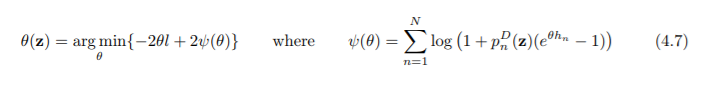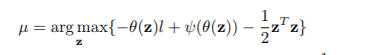## Global variables in nested optimization problem

Hi,

I'm trying to solve a nested optimization problem with SAS/IML, specifically):I'm not sure how to handle the input vs global variables in this case (which I think is why it is not working). I don't know how to handle z.  This is what i have so far:

/* function P*/
start P(z) global(h,b,p1,p2,a_inter,X_I);
return( sum(h#(CDF('NORMAL',(b-p1#(X_I*(a_inter*(z`))))/p2))) );
finish;

/* derivative of theta function*/
start deri(theta_x) global(l,h,z);
return( l - sum(P(z)#h#exp(h*theta_x)/( P(z)#(exp(h*theta_x)-1) + 1 ) ) );
finish;

/* finding root of theta function*/
start theta(z);
bounds = {0,10000};
return( froot( "deri", bounds) );
finish;

/*main function*/
start Func(z) global(l,h);
return( -theta(z)*l + (sum(log(1 + P(z)#(exp(h#theta(z)) - 1) ) )) - 0.5*z*(z`) );
finish;

z = RANDNORMAL(S,0, 1); /*x0*/
z = (z`);
opt = {1,2};
call nlpnra(rc, result, "Func", z, opt);

Given that the equations are done correctly, how would I set this up so that the root/derivative function can make use if the current z that the optimization algorithm is evaluating? Is there something else that is wrong?

1 ACCEPTED SOLUTION

Accepted Solutions

## Re: Global variables in nested optimization problem

You haven't defined S, l, or h.

I suggest you start by reading the article "Ten tips before you run an optimization." You also might want to look at "Optimizing a function of an integral," which optimizes a function that in turn calls other functions.

4 REPLIES 4

## Re: Global variables in nested optimization problem

You haven't defined S, l, or h.

I suggest you start by reading the article "Ten tips before you run an optimization." You also might want to look at "Optimizing a function of an integral," which optimizes a function that in turn calls other functions.

## Re: Global variables in nested optimization problem

I should have shown the step where i define them, my bad. (They are all vectors of length 1xN)

## Re: Global variables in nested optimization problem

Haven't looked through your functions in depth, but have have a few parameters, which you have not defined yet. Eg S and I.

Also, why do you set z = (z`) ?

## Re: Global variables in nested optimization problem

Sorry for the confusion, i should have shown the step where I import the other variables, all of them are defined vectors (1xN)

When trying the function one by one it seemed as z was transposed when i sent it in so i just did that as a quick fix.

From The DO Loop